## Algebra

Showing posts with label center. Show all posts
Showing posts with label center. Show all posts

### Circles

A circle is the set of points equidistant from a fixed point called the center. The distance from the center to any point is called the radius.  Recall the following formulas from Geometry: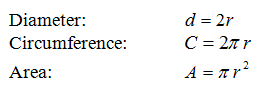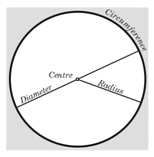The circumference can be thought of as the perimeter of the circle.  In this section, we will be interested in the equations that describe circles.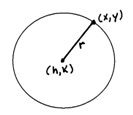From the equation of a circle in standard form we can see that a circle is completely determined by its center and radius.  In addition, notice that it is not a function because it fails the vertical line test.
[ InteractiveCircles ]

Given the equation of a circle in standard form, you can graph it using the following steps:
1. Plot the center (hk).
2. Use the radius r to plot four points up, down, left and right of the center.
3. Connect the points and label them.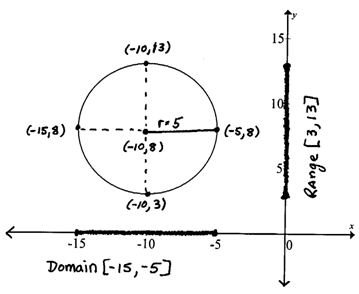Even though a circle is not a function, it is a relation and we could still find the domain and range.  In addition, we will be asked to find the x- and y-intercepts.  This particular example does not have any but the technique to find them is the same method used throughout this study guide.

To find y-intercepts set x = 0 and solve for y.
To find x-intercepts set y = 0 and solve for x.

Graph the circles and label the x- and y-intercepts.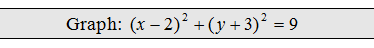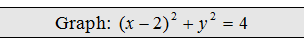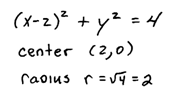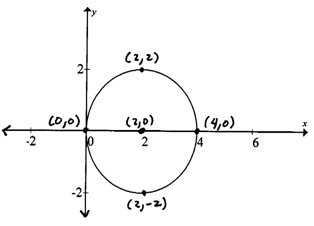Graphing circles in standard form is just a matter of identifying the center and the radius.  The difficulty comes when the circle is not given in standard form.  In this case, when given general form, we will complete the square twice as illustrated below.
1. Group all terms with variable x and group all terms with variable y.
2. Complete the square for each grouping.
3. Be sure to add (b/2)^2 to both sides of the equation.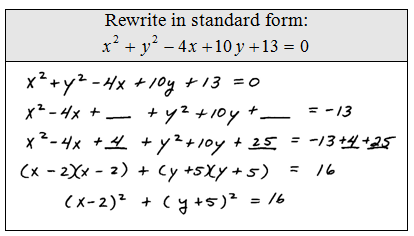Rewrite the circles in standard form and identify the center.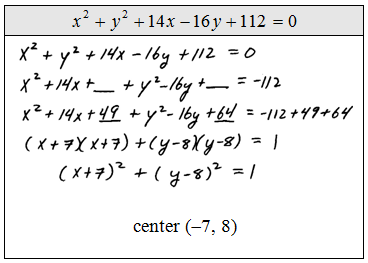Find the area of the following circles.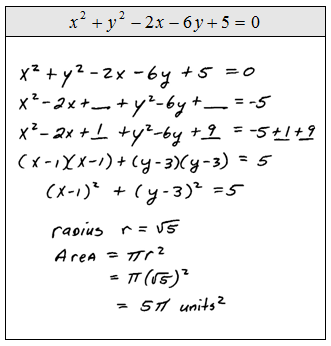Graph and find the x- and y-intercepts, area, and circumference.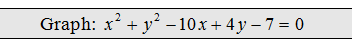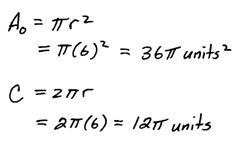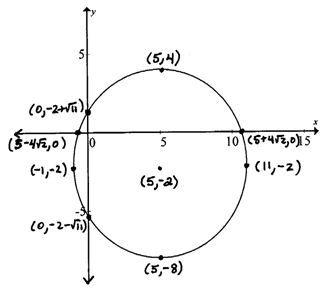Given the center and radius, find the equation of the circle.### Interactive: Circles

Interactive Instructions: Move the green points C and P. You can also drag the circle around.

[ NOTESCircles ]